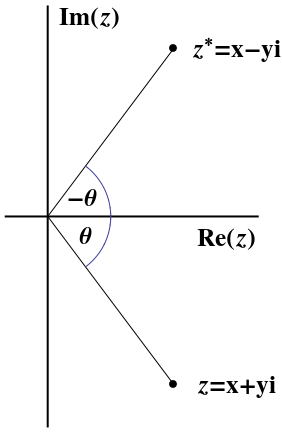The complex conjugate $z^*$ of a complex number $z=x+iy$ is found by replacing every $i$ by $-i\text{.}$ Therefore $z^*=x-iy\text{.}$ (A common alternate notation for $z^*$ is $\bar{z}\text{.}$) Geometrically, you should be able to see that the complex conjugate of ANY complex number is found by reflecting in the real axis, as shown in Figure 1.2.1.Figure 1.2.1. The point $z=x+iy$ and its complex conjugate $z^*=x-iy\text{.}$
Notice that this product is ALWAYS a positive, real number. The (positive) square root of this number is the distance of the point $z$ from the origin in the complex plane. We call the square root the norm or magnitude of $z$ and we use the same notation as "absolute value," i.e. $\vert z\vert\text{.}$ In this way, we see that the definition of absolute value, as in $\vert -2\vert=2\text{,}$ was never "strip off the minus sign," but really "how far is $-2$ from the origin.